# Test chart suitability for MTF measurements

 Related pages: Chart Quality Calculator – determine the suitability of a chart (based on MTF measurements for specific media & print methods) for a specific application

Whenever a test chart is used to measure camera MTF (sharpness)— and that includes a large portion of Imatest measurements— we need to know if the chart is good enough to reliably measure MTF. “Good enough” means that chart MTF projected on the image sensor will have minimal effect on the MTF measurement (which, by definition, includes the chart and the camera). This is generally the case for charts large enough to conform to traditional Imatest recommendations. But there are situations where the charts are pushed beyond their recommended limits, especially where space is limited, and we need to know how well the chart performs in these conditions. If the limits are not pushed too hard, you may be able to apply MTF compensation.

This page contains Chart MTF suitability displays for several standard test chart media– inkjet, photographic paper (reflective), and photographic film (transmissive).

 Implicit assumption on lens sharpness— What to do when sharpness is limited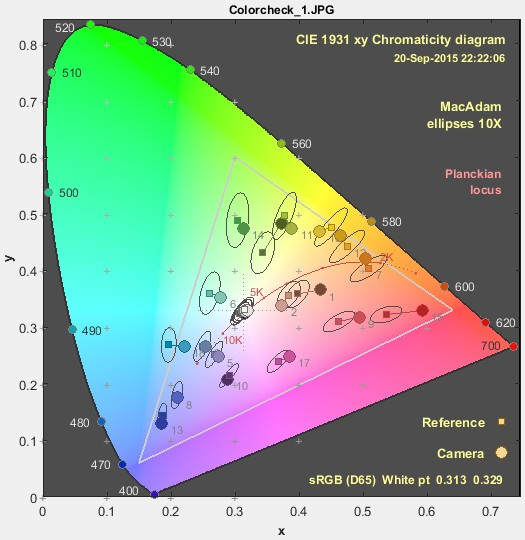CIE 1931 chromaticity diagram showing colors & wavelengths The calculations on this page implicitly assume that the lens is capable of producing sharp images at the pixel level, i.e., that it has the potential to be sharp, even if it doesn’t actually reach its potential. Unless we have reason to believe otherwise, we normally ignore this assumption. But if a lens is incapable of producing a sharp image, chart requirements can be relaxed.  What do we mean by “sharp image at the pixel level”? Based on over a decade of experience, my (NLK’s) estimate is that a “sharp” lens is capable of reaching an MTF of 0.3 (30%) at the Nyquist frequency, fNyq = 0.5 cycles/pixel. Lens sharpness is limited by aberrations, which can be controlled in high quality lenses, and by diffraction, which is a fundamental physical limit resulting from the wave nature of light. The cutoff frequency for diffraction is $$f_{\mathit{cutoff}} = 1/(\lambda N)$$, where λ is the wavelength, typically 555 nm = 0.000555 mm (yellow-green; around the wavelength where the eye is most sensitive) and N is the lens f-stop (F-number).  For the vast majority of lenses, the diffraction limit is well above fNyq, and can be ignored in test chart suitability calculations. But there are some new sensors (for example the 108MP Samsung 108MP ISOCELL HM2 with 0.7 micron pixels), where this may not hold. In developing the procedure for dealing with diffraction, we note that the relative frequencies where diffraction-limited MTF equals specified values (fnn for MTF = nn%) are a simple fractions of $$f_{\mathit{cutoff}} =1/(\lambda N)$$ $$f_{90} = 0.0786 /(\lambda N); \ \ \ \ \ f_{70} = 0.237/(\lambda N); \ \ \ \ \ f_{50} = 0.404/(\lambda N); \ \ \ \ \ f_{30} = 0.585 /(\lambda N)$$ For pixel size p,  $$f_{Nyq} = 1/(2p)$$ Relaxing test chart requirements— If $$f_{30} < f_{Nyq}$$, multiply Sensor height in pixels (the left y-axis in the figures below) by $$f_{30} / f_{Nyq}$$. This decreases the recommended chart size. If $$f_{30} > f_{Nyq}$$, leave it unchanged. See Example 2, below, for the 108MP ISOCELL HM2 sensor.

The following table is based on experience using chart compensation, where the key metric that determines chart quality is the projected chart MTF on the image sensor at the Nyquist frequency, fNyq = 0.5 cycles/pixel.

 MTF @ fNyq Quality Description MTFNyq ≥ 0.90 Excellent Chart compensation is unnecessary; will not improve accuracy. 0.70 ≤ MTFNyq < 0.90 Good Chart compensation is recommended but optional. It improves accuracy. MTFNyq = 0.77 corresponds to the traditional Imatest chart size recommendation. 0.50 ≤ MTFNyq < 0.70 Comp. required Chart compensation is required for accurate results. 0.30 ≤ MTFNyq < 0.50 Minimal Will work with chart compensation, but this is stretching things.  Not recommended. MTFNyq < 0.30 Not usable Don’t even think of it!  MTF is too low for reliable results (it’s too sensitive to noise), even with compensation.

#### Chart MTF Suitability display

This display, calculated in the Chart Quality Calculator in Imatest 5.2+, is recommended for determining the suitability of a chart for MTF measurements for a given camera system.

You don’t need to have Imatest installed to use the Chart MTF Suitability display results on this page.

It is based on the concept that only three items (one file and two parameters) are needed to determine chart suitability.

1. the chart compensation file, which has a model of the chart MTF for a specific print technology (inkjet, photo paper, photo film, etc.)
2. the vertical field of view (VFoV)
3. the vertical pixel count of the sensor (Vpix)

If you have the chart compensation file and know VFoV and Vpix, you can easily determine chart suitability from the Chart Suitability display. All other parameters, such as angular field of view, lens-to-chart distance, sensor height, and pixel pitch, are only used to calculate items 2 and 3.

Note that this approach only works for rectilinear (low barrel distortion) images. It has to be modified for highly barrel-distorted (fisheye) lenses. Horizontal may be substituted for Vertical dimensions as long as consistency is maintained.

To use this display in Imatest, read the chart compensation file, then press Chart MTF suitability display at the bottom of the Chart quality calculator window. The box near the top with descriptions of the media is for annotation-only. The aspect ratio, used for the megapixel scale on the right y-axis, can be set to 4:3, 3:2, or 16:9 in the small dropdown menu on the right.

This page has results for typical samples of a number of printing technologies.

 Using the Chart suitability figures Determine the vertical pixel count from the image itself or the image sensor specification. This is Sensor height in pixels on the left y-axis.    Note that Megapixels on the right y-axis for the selected aspect ratio (3:2 is shown below) corresponds to Sensor height in pixels  on the left y-axis.   The Vertical Field of View (V-FoV) in centimeters is shown on the x-axis. It is typically slightly larger than the height of charts designed to fill the frame (SFRplus ,and eSFR ISO, and Checkerboard). Note that Spilled Coins, Siemens Star, and Log F-Contrast) are not designed to fill the frame in high resolution cameras— they should occupy no more than about 2000 vertical pixels. For these charts, V-FoV is often much larger than the chart height.   Diagonal lines represent MTF@fNyq for the chart image projected on the sensor (the result of the measurement described in Compensating Camera MTF Measurements). You don’t need to know the details to use these charts.    Colors correspond to the colors on the Quality table (above), i.e., green (lower-right) is excellent; yellow requires MTF compensation, pink (upper-left) is unusable, etc. (The Matlab exponential notation on the x and y-axes is not my favorite: 100 = 1; 101 = 10; 102 = 100, etc.)   For the Sensor height in pixels, choose the required quality level, then find the recommended V-FoV (object height) corresponding to the diagonal line for the quality level. Or choose a chart size and find where the corresponding vertical line interescts the horizontal line for Sensor height in pixels. Example 1:  For a sensor height of 3000 pixels, which corresponds to 13.5 MP for a 3:2 aspect ratio, and for a matte inkjet chart (below), Excellent quality (no compensation necessary) corresponds to Vertical Field-of-View (V-FoV) = 110 cm.  Imatest’s traditional recommendation (computation optional) is V-FoV = 54 cm. Minimum recommended V-FoV(compensation required) = 27 cm.   Example 2:  For the 108 MP (pixel height = 9000) Samsung ISOCELL HM2 with 0.7 micron pixels) with an f/2 aperture (N = 2), Nyquist frequency fNyq = 1/(2×0.0007mm) = 714.3 cy/mm. The cutoff frequency is  fcut = 1/(λN) = 900.9 cycles/mm, but the frequency where MTF = 0.3 (30%) is  f30 = 0.585/(λN) = 527.0 cy/mm, which is well below the Nyquist frequency.  In this case,  f0.3 / fNyq = 0.738, so use 0.738×9000 = 6642 as Sensor height in pixels.  The (corrected) traditional recommendation for V-FoV (chart height) is 100 cm vs. 160 cm without the correction.

### Reflective charts

#### Inkjet matte surface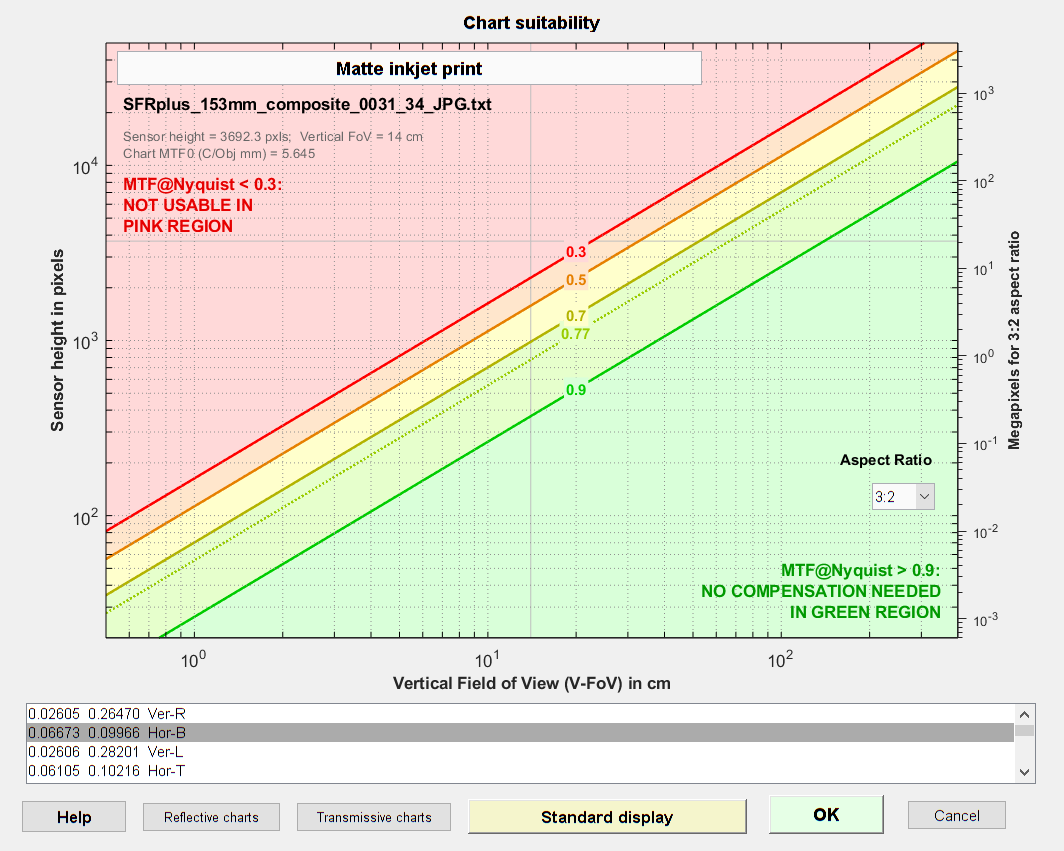Matte inkjet film Chart Suitability display.  Click on the image to display full-sized.
Good_Inkjet_2019_DSC00074_JPG.txt

#### B&W photographic paper charts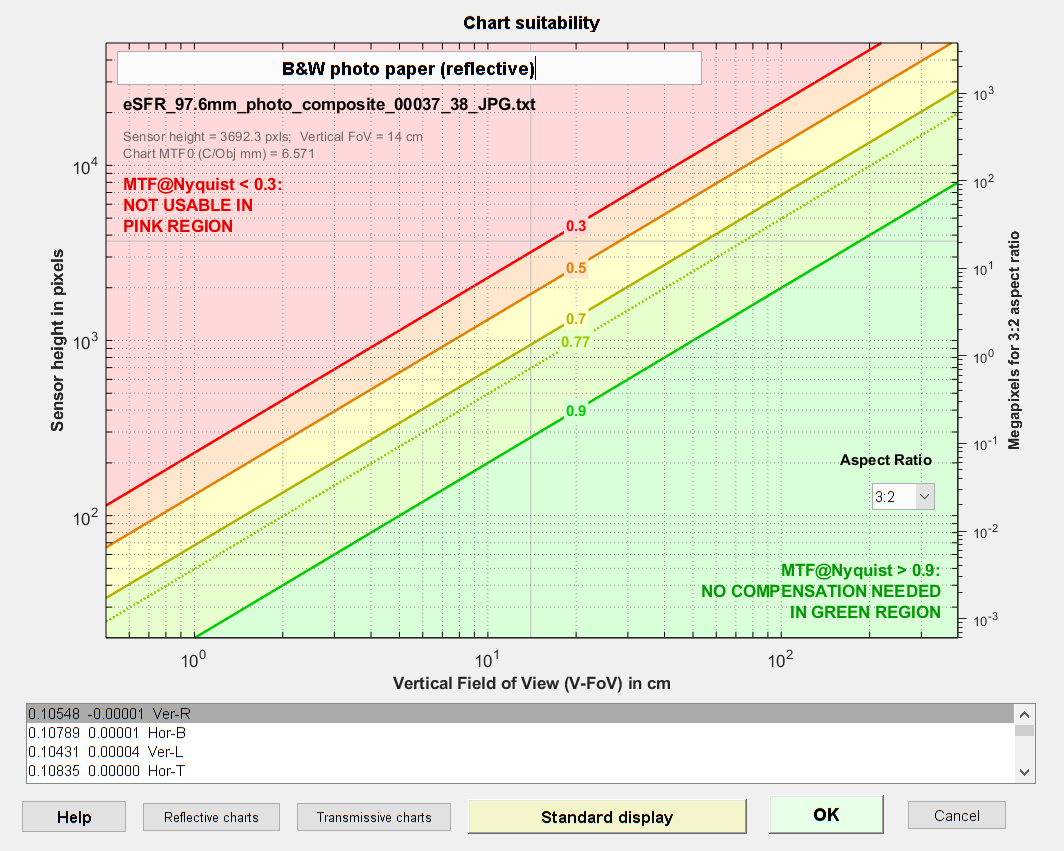Black & White LVT film Chart Suitability display.  Click on the image to display full-sized.

### Transmissive (backlit) charts

#### Color LVT film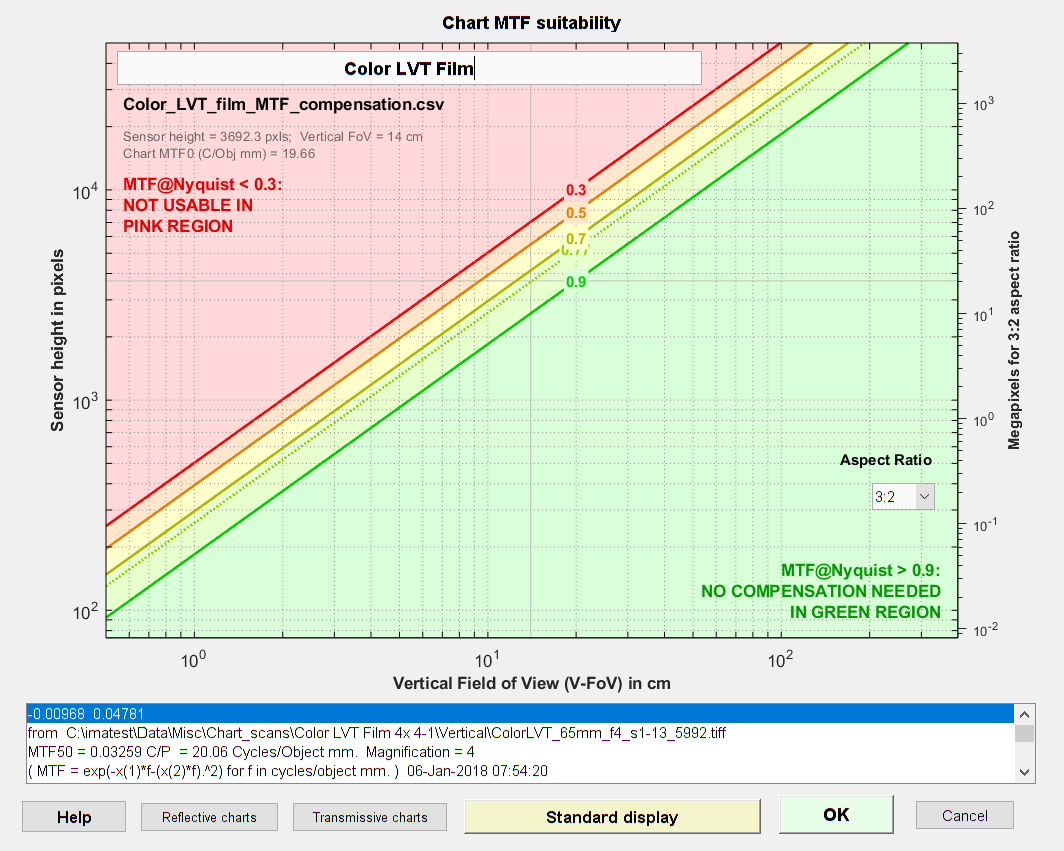Color LVT film Chart Suitability display.  Click on the image to display full-sized.

####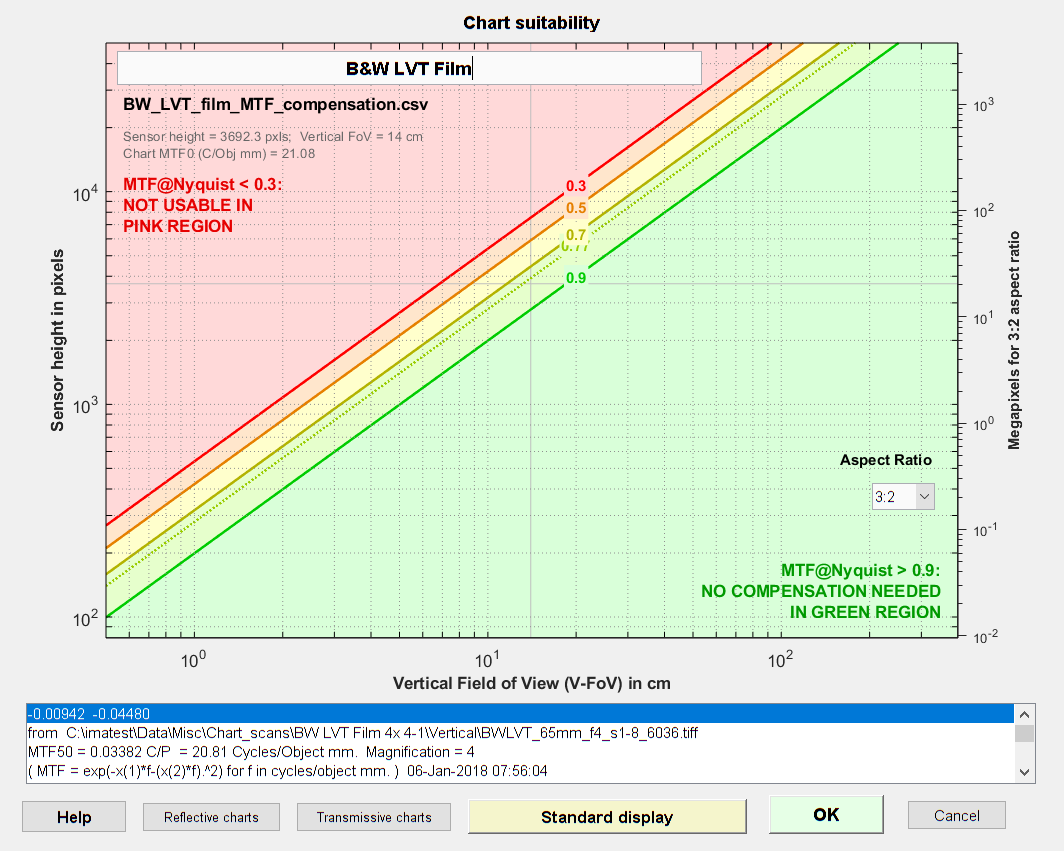Black & White LVT film Chart Suitability display.  Click on the image to display full-sized.

Example using B&W LVT film: The standard Black & White LVT chart, printed on 12×20 inch film, is designed to have a 27 cm vertical Field of View. 27 cm (on the x-axis) corresponds to MTF@Nyquist = 0.9 (the green diagonal line, below) for sensor height = 5500 pixels (left y-axis) or 45 megapixels at 3:2 aspect ratio (right y-axis). At this high quality level, MTF compensation is not required. If we push the chart to MTF@Nyquist = 0.7 (the olive diagonal line), which is still reasonably good, but requires MTF compensation, we reach sensor height = 8500 pixels (about 100 Megapixels at 3:2 aspect ratio).

### Appendix: Chart MTF and suitability calculations

Chart MTF is measured using printed slanted-edges of reasonable contrast (between 4:1 and 10:1) with the technique described in the Appendix of Compensating Camera MTF Measurements. The spatial frequency unit for the chart MTF measurement is cycles/object mm, i.e., cycles per millimeter on the chart (not on the sensor, as is typical of most Imatest measurements). Here are a few details about the measurement (more in the Appendix).

• Magnification should be large enough (typically around 0.5x for inkjet charts and 1x for photographic charts) so that the measurement is dominated by the chart quality, not by the lens, sensor, or signal processing. (Typical camera MTF measurements have magnifications of < 0.1 for high quality film charts and < 0.02 for inkjet charts.) Magnification should be carefully measured and recorded.
• The measured chart MTF is fit to a function that closely approximates actual response curves at frequencies where MTF > 0.3 (below MTF30). This function removes irregularities in the MTF curve caused by noise (typically at frequencies above MTF30) and facilitates further processing. The function is

$$\displaystyle MTF_{chart}(f) = e^{-a_1 f – (a_2 f)^2}$$ ;         f in units of Cycles/Object mm (C/Obj mm).

This function matches a wide range of observed chart MTF measurements.

#### Projected Chart MTF

When the chart is projected (imaged) on the sensor its spatial frequency units must be transformed to the native units of the sensor, Cycles/Pixel (C/P), by substituting,

$$f(\text{C/Obj mm}) = f(C/P) \times \text{magnification} \times \text{pixels/mm}$$

$$MTF_{chart-projected} (f) = MTF_{chart}(f(C/P) \times \text{magnification} \times \text{pixels/mm}) = MTF_{div}(f)$$

In Imatest sharpness modules, pixels/mm is typically derived from the user-entered value of pixel pitch (in μm), where pixels/mm = 1000 / (pixel pitch (μm)). Magnification is either entered in the settings window or derived from geometrical factors (like the bar-to-bar spacing in SFRplus or the vertical registration mark spacing in eSFR ISO).

If MTFdiv(fNyq) (MTFdiv at the Nyquist frequency (fNyq = 0.5 C/P)) is lower than 0.9 (90%), measurement accuracy can be improved by dividing the measured MTF by MTFdiv, as described in Compensating camera MTF measurements for chart and sensor MTF. If MTFdiv(fNyq) is greater than 0.9, MTF compensation offers little improvement.

Note that for rectilinear images (not strongly barrel distorted, i.e., not fisheye),

$$\text{magnification} = \text{sensor height} / \text{Vertical FoV}$$    and

$$\text{pixels/mm} = \text{Vertical pixels} / \text{sensor height}$$

Therefore we can say

$$f(\text{C/Obj mm}) = f(C/P) \times \text{Vertical pixels} / \text{Vertical FoV}$$

This formulation is used in the Chart Suitability display.

Fisheye (strongly barrel-distorted) lenses present a problem because their magnification decreases with distance from the image center. To use the Chart Suitability display, we have to create a sort of “virtual Vertical FoV” based on magnification at the center. Fortunately this isn’t too difficult because fisheye lenses are rarely used for extreme closeup images (except in medical endoscopes). In most cases the lens-to-chart distance dlens-chart >> lens focal length f, and hence we can use magnification = f / dlens-chart = Vertical FoV/sensor height.

$$\text{virtual Vertical Field of View} = VVFoV = \text{magnification} \times \text{sensor height}$$

VVFoV can be significantly larger than the actual physical FoV.